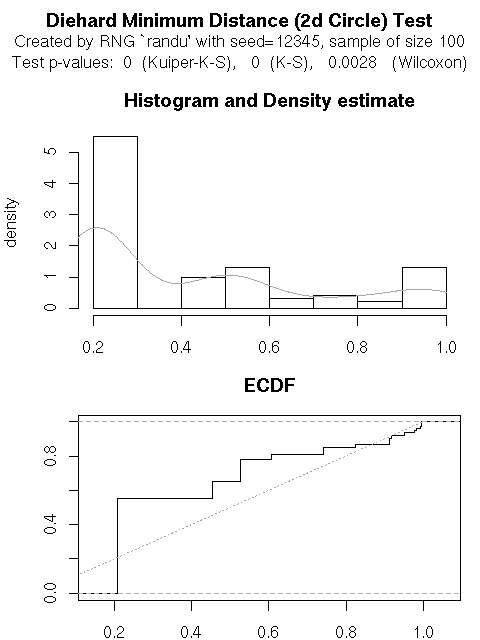## Excecutive Summary

RDieHarder is a fairly straightforward 'port' of the dieharder command-line functionality to GNU R. It gives R access to Robert G. Brown's dieharder test suite for random number generators, which is itself a rewrite and extension of Marsaglia's older diehard test battery. In the process, we also ported R's (currently six) random number generators to the dieharder framework of wrapping over sixty generators from the GNU GSL.

## Rationale and possible extensions

By using GNU R to further analyse and visualize the test results, we hope to help with development of further tests statistics for RNGs, as well as to help with new or different analysis of the existing test statistics.

Future goals are to also allow for

• analysis of user-contributed RNGs that are supplied via R's interface
• analysis of parallel RNGs such as SPRNG and some of Pierre L'Ecuyer's parallel generators (both of which R can use for parallel / high-erformance computing)
• analysis of other generators accessible to R as e.g. the AES generator packaged by Thomas Lumley.
I am grateful for comments by Luke Tierney as the discussant and others at UseR! 2007 for some of these suggestions.

## Example session

Usage is as simple as loading the package and calling the `dieharder` function:
```> library(RDieHarder)
> dhtest <- dieharder(rng="randu", test=10, psamples=100, seed=12345)
```
We can now call the `print` method
```> print(dhtest)

Diehard Minimum Distance (2d Circle) Test

data:  Created by RNG `randu' with seed=12345, sample of size 100
p-value < 2.2e-16

```
This shows that the 'randu' generator (which is known as one of the worst available ones and should be avoided at almost all cost) fails the minimum-distance / 2dsphere test.

The `summary` method shows more detail, including a simple stem plot as an alternative to the histogram shown by dieharder's command-line tool. This stem plot, as well as the summary statistics, clearly show how degenerate the results are for this test statistics with the minimum to median p-value being 0.2086 we are clearly not seeing a uniform distribution over the range from zero to one:

```> summary(dhtest)

Diehard Minimum Distance (2d Circle) Test

data:  Created by RNG `randu' with seed=12345, sample of size 100
p-value < 2.2e-16

Summary for test data
Min. 1st Qu.  Median    Mean 3rd Qu.    Max.
0.2086  0.2086  0.2086  0.4173  0.5271  0.9956

Stem and leaf plot for test data

The decimal point is 1 digit(s) to the left of the |

2 | 1111111111111111111111111111111111111111111111111111111
3 |
4 | 6666666666
5 | 3333333333333
6 | 111
7 | 4444
8 | 22
9 | 111225588999
10 | 0

NULL

One-sample Kolmogorov-Smirnov test

data:  object\$data
D = 0.3414, p-value = 1.512e-10
alternative hypothesis: two-sided

Wilcoxon signed rank test with continuity correction

data:  object\$data
V = 1675, p-value = 0.002847
alternative hypothesis: true location is not equal to 0.5

Warning message:
cannot compute correct p-values with ties in: ks.test(object\$data, "punif", 0, 1, exact = TRUE)

```
where we even get warning for ties among the hundred p-values. Lastly, a `plot` plot methods provides the histogram and ecdf of the p-values used for the aggregate test statistics and creat the chart below (where we clearly see the non-uniform distribution of test statistics leading tothe clear rejection of this test:```      svn checkout http://rdieharder.googlecode.com/svn/trunk/ rdieharder